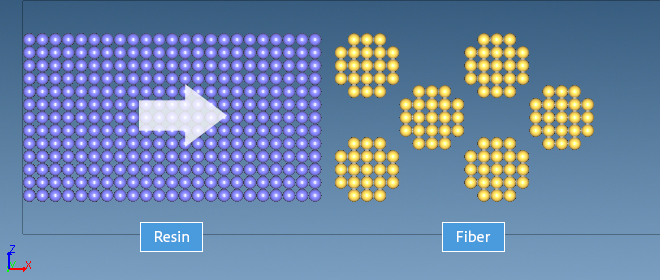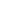Continuum model
Other Properties
Materials Science

# [Analysis Example] Resin impregnation process calculation for fiber structure by VSOP-PS

Process simulation using Particle method

## Objectives and Methods

Carbon fiber reinforced plastics (CFRP) and thermoplastic carbon fiber reinforced plastics (CFRTP) have high strength and light weight and are used for aircraft and automotive parts and concrete reinforcement. On the other hand, the resin impregnation molding process of these materials has a problem that residual voids affect their strength. This case study presents an example of void formation calculation in the resin impregnation process for fiber structures.

VSOP-PS, one of the engines of J-OCTA, solves the Navie-Stokes equations using the particle method (MPS = Moving Particle Simulation (or Semi-Implicit method)). Compared to other methods, this method has the advantage of easy handling of free surfaces. The model consists of a fiber and a resin, each made of particles, as shown in Figure 1. The fiber binds the particles together and constrains their positions. The resin permeates the fiber by applying pressure in the x direction. The calculation is 3-dimensional and the boundary conditions are periodic boundaries in each direction.Figure1 : Resin penetration model

## Results

Figure 2 shows the difference in the formation of voids between fibers when the interfacial tension between fibers and resin is changed in the resin-fiber penetration calculation modeled in Figure 1. In the left figure, when the interfacial tension is strong, the resin is densely distributed between the fibers. On the right, when the interfacial tension is weak, voids are formed between fibers. The calculation time is about 30 minutes to 2 hours (about 5000 particles).Figure2 : Shows the difference in the formation of voids between fibers when the interfacial tension between fibers and resin is changed